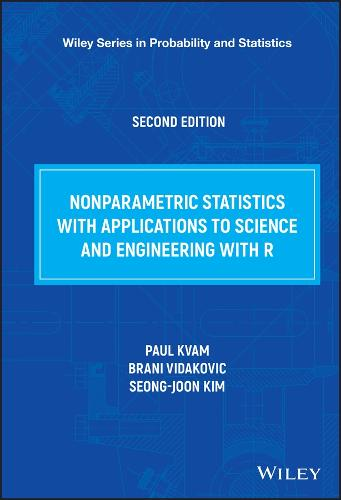•# Nonparametric Statistics with Applications to Science and Engineering - Wiley Series in Probability and Statistics (Hardback)

(author), (author)
£112.00
Hardback 430 Pages / Published: 20/02/2019
• We can order this

Usually dispatched within 1 week

This book presents modern nonparametric statistics from a practical point of view. This new edition includes custom R functions implementing nonparametric methods to explain how to compute them and make them more comprehensible. Relevant built-in functions and packages on CRAN are also provided with a sample code. R codes in the new edition not only enable readers to perform nonparametric analysis easily, but also to visualize and explore data using R's powerful graphic systems, such as ggplot2 package and R base graphic system. Following an introduction and a discussion of the basics of probability, statistics, and Bayesian statistics, the book discusses order statistics, Kolmogorov-Smirnov test statistic, rank tests, and designed experiments. Next, categorical data, estimating distribution functions, and density estimation is examined. Least squares regression is covered, along with curve fitting techniques, wavelets, and bootstrap sampling. Other topics examined include EM algorithm, statistical learning, nonparametric Bayes, and WinBUGS. This book will be of interest to graduate students in engineering and the physical and mathematical sciences as well as researchers who need a more comprehensive, but succinct understanding of modern nonparametric statistical methods.

Publisher: John Wiley & Sons Inc
ISBN: 9781119268130
Number of pages: 430
Weight: 666 g
Edition: 2nd Edition